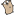## Go by Example: Constants and iota

 Go supports constants of character, string, boolean, and numeric values. We can use iota to simulate C’s enum or #define constant.```package main ``` ```import ( "fmt" "strconv" ) import "math" ``` `const` declares a constant value. ```const s string = "constant" ``` Sepcial method to generate maximum of uint. Ref: http://blog.golang.org/constants ```const MaxUint = ^uint(0) const MaxUint32 = ^uint32(0) ``` Here we simulate C’s `enum` by Go’s `iota` ```type Season uint8 ``` ```const ( Spring = Season(iota) Summer Autumn Winner ) ``` output function for Season variable ```func (s Season) String() string { name := []string{"spring", "summer", "autumn", "winner"} i := uint8(s) switch { case i <= uint8(Winner): return name[i] default: return strconv.Itoa(int(i)) } } func main() { fmt.Println(s) ``` A `const` statement can appear anywhere a `var` statement can. ``` const n = 500000000 ``` Constant expressions perform arithmetic with arbitrary precision. ``` const d = 3e20 / n fmt.Println(d) ``` A numeric constant has no type until it’s given one, such as by an explicit cast. ``` fmt.Println(int64(d)) ``` A number can be given a type by using it in a context that requires one, such as a variable assignment or function call. For example, here `math.Sin` expects a `float64`. ``` fmt.Println(math.Sin(n)) ``` Assign Season variable and print it ``` s := Summer fmt.Println(s) ``` Assign invalid range Season variable and print it ``` s = Season(9) fmt.Println(s) ``` ``` fmt.Println(MaxUint) fmt.Println(MaxUint32) } ```
 ```\$ go run constant.go constant 6e+11 600000000000 -0.28470407323754404 summer 9 18446744073709551615 4294967295 ```

Previous example: Variables.

Next example: For.